1. /
2. CBSE
3. /
4. Class 09
5. /
6. Mathematics
7. /
8. NCERT Solutions for Class...

# NCERT Solutions for Class 9 Maths Exercise 14.4

NCERT solutions for Class 9 Maths Statistics

## NCERT Solutions for Class 9 Mathematics Statistics

###### 1. The following number of goals were scored by a team in a series of 10 matches:

2, 3, 4, 5, 0, 1, 3, 3, 4, 3

Find the mean, median and mode of these scores.

Ans. We know that, Mean =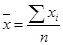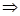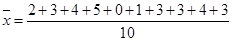=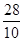= 2.8

For median,

Arrange the given data in the ascending order, we get

0, 1, 2, 3, 3, 3, 3, 4, 4, 5

Herewhich is even in number.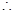Median =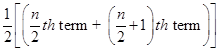Median =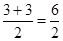= 3

For mode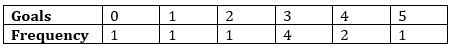Here, Goal – 3 has the maximum frequency is 4. Therefore, mode = 3

NCERT Solutions for Class 9 Maths Exercise 14.4

###### 2. In a mathematics test given to 15 students, the following marks (out of 100) are recorded:

41, 39, 48, 52, 46, 62, 54, 40, 96, 52, 98, 40, 42, 52, 60

Find the mean, median and mode of this data.

Ans. We know that, Mean =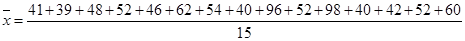=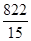= 54.8

For median,

Arrange the given data in the ascending order, we get

39, 40, 40, 41, 42, 46, 48, 52, 52, 52, 54, 60, 62, 96, 98

Here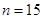which is odd in number.Median =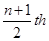term =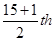term = 8th observationMedian = 52Median == 3

For mode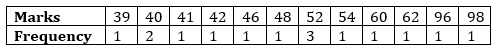Here, Marks – 52 has the maximum frequency is 3. Therefore, mode = 52

NCERT Solutions for Class 9 Maths Exercise 14.4

###### 3. The following observations have been arranged in the ascending order. If the median of the data is 63, find the value of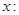29, 32, 48, 52,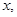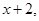72, 78, 84, 95

Ans. Here number of observations = 10, which is an even numberMedian ==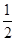(5th term + 6th term)63 =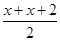63 =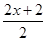63 =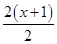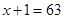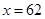NCERT Solutions for Class 9 Maths Exercise 14.4

###### 4. Find the mode of the following data in each case:

(i) 14, 25, 14, 28, 18, 17, 18, 14, 23, 22, 14, 18

(ii) 7, 9, 12, 13, 7, 12, 15, 7, 12, 7, 25, 18, 7

Ans. (i) Making a frequency table, we have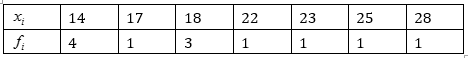Here the observation 14 has the maximum frequency 4.

Therefore, mode = 14

(ii) Making a frequency table, we have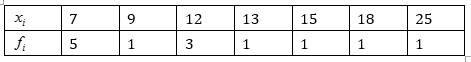Here the observation 7 has the maximum frequency 5.

Therefore, mode = 7

NCERT Solutions for Class 9 Maths Exercise 14.4

###### 5. Find the mean salary of 60 workers of a factory from the following table: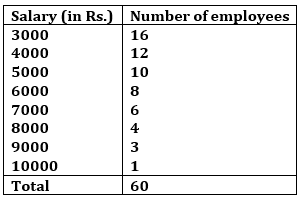Ans.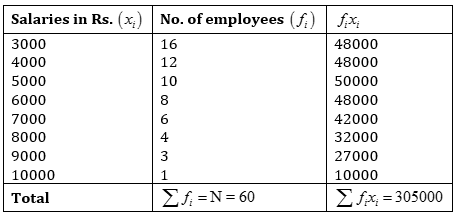Mean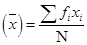=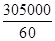= 5083.33

Hence the mean of salary is Rs. 5083.33

NCERT Solutions for Class 9 Maths Exercise 14.4

###### 6. Give an example of a situation in which:

(i) the mean is an appropriate measure of central tendency.

(ii) the mean is not an appropriate measure of central tendency but the median is an appropriate measure of central tendency.

Ans. (i) Mean is the suitable measure of central tendency because every term is taken in

calculation, it is effected by every item. It can further be subjected to algebraic treatment unlike other measures i.e. median and mode. As mean is rigidly defined, it is mostly used for comparing the various issues.

For example, the marks obtained by the seven students are 10, 15, 14, 18, 26, 24, 20, 14 and 27.Mean =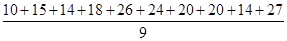=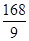= 18.67

But median of 10, 15, 14, 18, 26, 24, 20, 14 and 27 is 18 and mode is 14.

###### From above example we conclude that mean is rigidly defined and the marks 18.67 represent the performance of 9 students, but median and mode are not so rigidly defined.

(ii) (a) Mean is much affected by the extreme value but median is not much affected by these

extremes.

For example, if there are 5 terms: 4, 7, 12, 18, 19

Mean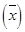is 12 in this case and median is 12.

If we add two values 450 and 1000 the new mean =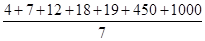=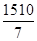= 215.7

This is a big change as compared to the size of first five terms’ mean but new median of 4, 7, 12, 18, 19, 450, 1000 is 18 which is not so much changed as compared to the size of first five terms’ median.

So we conclude that the mean misrepresents the result if extreme values are present in the data.

(b) Sometimes mean gives impossible conclusion e.g. if there are 60, 50 and 42 students in three classes then mean number of students is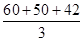= 50.67, which is impossible as students cannot be in fractions. But median of 42, 50 and 60 is 50.

## NCERT Solutions for Class 9 Maths Exercise 14.4

NCERT Solutions for Class 9 Maths PDF (Download) Free from myCBSEguide app and myCBSEguide website. Ncert solution class 9 Maths includes text book solutions from Mathematics Book. NCERT Solutions for CBSE Class 9 Maths have total 15 chapters. 9 Maths NCERT Solutions in PDF for free Download on our website. Ncert Maths class 9 solutions PDF and Maths ncert class 9 PDF solutions with latest modifications and as per the latest CBSE syllabus are only available in myCBSEguide.

## CBSE app for Class 9

To download NCERT Solutions for Class 9 Maths, Computer Science, Home Science,Hindi ,English, Social Science do check myCBSEguide app or website. myCBSEguide provides sample papers with solution, test papers for chapter-wise practice, NCERT solutions, NCERT Exemplar solutions, quick revision notes for ready reference, CBSE guess papers and CBSE important question papers. Sample Paper all are made available through the best app for CBSE students and myCBSEguide website.### Test Generator

Create question paper PDF and online tests with your own name & logo in minutes.### myCBSEguide

Question Bank, Mock Tests, Exam Papers, NCERT Solutions, Sample Papers, Notes

### 10 thoughts on “NCERT Solutions for Class 9 Maths Exercise 14.4”

1. it is useful for me thanks

2. it is useful for me

3. Thankyou so much

4. thank you i like it

5. Thanks

6. I love you mycbseguide

7. Thank you

8. Thank you so much

9. Like it bro it is good and

10. Wow just an interesting website from which we can do any homework and also can understand any question……….